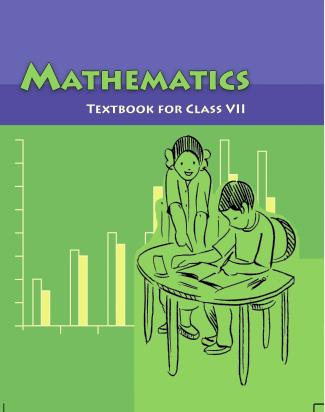1. /
2. CBSE
3. /
4. Class 07
5. /
6. Mathematics
7. /
8. NCERT Solutions for Class...

# NCERT Solutions for Class 7 Maths Exercise 1.2### myCBSEguide App

Download the app to get CBSE Sample Papers 2023-24, NCERT Solutions (Revised), Most Important Questions, Previous Year Question Bank, Mock Tests, and Detailed Notes.

NCERT solutions for Class 7 Integers Maths## NCERT Solutions for Class 7 Maths Integers

###### Question 1.Write down a pair of integers whose:

(a) sum is {tex} – 7{/tex}

(b) difference is {tex} – 10{/tex}

(c) sum is 0

(a) One such pair whose sum is {tex} – 7{/tex}: {tex} – 5 + \left( { – 2} \right) = – 7{/tex}

(b) One such pair whose difference is {tex} – 10{/tex}: {tex} – 2 – 8 = – 10{/tex}

(c) One such pair whose sum is 0: {tex} – 5 + 5 = 0{/tex}

NCERT Solutions for Class 7 Maths Exercise 1.2

###### Question 2.(a) Write a pair of negative integers whose difference gives 8.

(b) Write a negative integer and a positive integer whose is {tex} – 5.{/tex}

(c) Write a negative integer and a positive integer whose difference is {tex} – 3.{/tex}

(a) {tex} – 2 – \left( { – 10} \right) – 2 + 10 = 8{/tex}

(b) {tex}\left( { – 7} \right) + 2 = – 5{/tex}

(c) {tex}\left( { – 2} \right) – 1 = – 2 – 1 = – 3{/tex}

NCERT Solutions for Class 7 Maths Exercise 1.2

###### Question 3.In a quiz, team A scored {tex} – 40,10,0{/tex} and team B scores 10, 0, {tex} – 40{/tex} in three successive rounds. Which team scored more? Can we say that we can add integers in any order?

Team A scored {tex} – 40,10,0{/tex}

Total score of Team A = {tex} – 40 + 10 + 0 = – 30{/tex}

Team B scored {tex}10,0, – 40{/tex}

Total score of Team B = {tex}10 + 0 + \left( { – 40} \right) = 10 + 0 – 40 = – 30{/tex}

Thus, scores of both teams are same.

Yes, we can add integers in any order due to commutative property.

NCERT Solutions for Class 7 Maths Exercise 1.2

###### Question 4.Fill in the blanks to make the following statements true:

(i){tex}\left( { – 5} \right) + \left( { – 8} \right) = \left( { – 8} \right) + \left( {…….} \right){/tex}

(ii){tex} – 53 + ……. = – 53{/tex}

(iii)17 + ……. = 0

(iv){tex}\left[ {13 + \left( { – 12} \right)} \right] + \left( {…….} \right) = 13 + \left[ {\left( { – 12} \right) + \left( { – 7} \right)} \right]{/tex}

(v){tex}\left( { – 4} \right) + \left[ {15 + \left( { – 3} \right)} \right] = \left[ { – 4 + 15} \right] + …….{/tex}

(i) {tex}\left( { – 5} \right) + \left( { – 8} \right) = \left( { – 8} \right) + \underline {\left( { – 5} \right)} {/tex} [Commutative property]

(ii) {tex} – 53 + \underline 0 = – 53{/tex} [Zero additive property]

(iii) {tex}17 + \underline {\left( { – 17} \right)} = 0{/tex} (Additive identity]

(iv){tex}\left[ {13 + \left( {12} \right)} \right] + \underline {\left( { – 7} \right)} = 13 + \left[ {\left( { – 12} \right) + \left( { – 7} \right)} \right]{/tex} [Associative property]

{tex}\left( { – 4} \right) + \left[ {15 + \left( { – 3} \right)} \right] = \left[ { – 4 + 15} \right] + \underline {\left( { – 3} \right)} {/tex} [Associative property]

## NCERT Solutions for Class 7 Maths Exercise 1.2

NCERT Solutions Class 7 Maths PDF (Download) Free from myCBSEguide app and myCBSEguide website. Ncert solution class 7 Maths includes text book solutions from Class 7 Maths Book . NCERT Solutions for CBSE Class 7 Maths have total 15 chapters. 7 Maths NCERT Solutions in PDF for free Download on our website. Ncert Maths class 7 solutions PDF and Maths ncert class 7 PDF solutions with latest modifications and as per the latest CBSE syllabus are only available in myCBSEguide.

## CBSE app for Students

To download NCERT Solutions for Class 7 Maths, Social Science Computer Science, Home Science, Hindi English, Maths Science do check myCBSEguide app or website. myCBSEguide provides sample papers with solution, test papers for chapter-wise practice, NCERT solutions, NCERT Exemplar solutions, quick revision notes for ready reference, CBSE guess papers and CBSE important question papers. Sample Paper all are made available through the best app for CBSE students and myCBSEguide website.### Test Generator

Create question paper PDF and online tests with your own name & logo in minutes.### myCBSEguide

Question Bank, Mock Tests, Exam Papers, NCERT Solutions, Sample Papers, Notes

### 4 thoughts on “NCERT Solutions for Class 7 Maths Exercise 1.2”

1. one mistake it is class not lass

2. Dude ur app has help us a lot .

3. Very nice

4. Ncert math 1.2 ka ans book me nahi mil raha hai